# Chemistry and Kinetics of TiCl4 Burner

Thermochemical simulation of reactive high temperature reaction mixtures were performed by combining overall reaction kinetics with the multi-component Gibbs energy minimisation. The mass and heat transfer conditions were combined with the enthalpy changes due to chemical reactions. Consequently, the change of temperature in the reactive system was calculated in terms of the extent of the overall reaction. ChemSheet was used as the modelling environment in this example, which shows a simulation of a titanium(IV) chloride burner, known for its wide industrial use in TiO2-pigment manufacture. It is important to note that same method can be applied for several other multi-component reactors.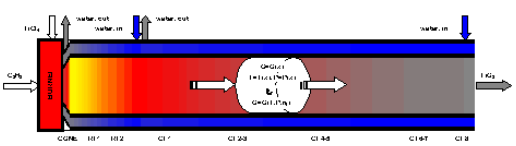Figure 1. The 1-dimensional model of the TiCl4-burner in ChemSheet.

The extent of reaction (ξr), the enthalpies of reaction and the heat exhange between the reaction mixture and the cooling water are combined into a steady state simulation in terms of the reactor axis (x). The key reactions and their data (at ca. 1000°C) are as follows:

 TiCl4(g) + O2(g) <-> TiO2(s) + 2Cl2(g) (I) Cl2(g) <-> 2Cl(g) (II)
 Reaction ΔH/kJmol-1 ΔG/kJmol-1 Ea/kJmol-1 I -177 -102 70 – 100 II 250 98 –

The activation energy Ea is deduced from the first order rate law of reaction (I), as determined by Pratsinis & al1: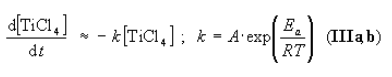with A = 8.26·104 s-1. The rates for chlorine association-dissociation reactions and other side reactions such as oxychloride formation are not known for the burner conditions. Thus, reaction (II) and other side reactions were assumed to be in equilibrium.

To construct the model, the engineers combined the reaction rate of equation (III) with the multi-component equilibrium calculation in ChemSheet. The temperature of the reaction mixture and the temperature of the cooling water on each step are received by applying a radial heat transfer model. The basic algorithm which combines the reaction kinetics with the thermochemical system is described in figure 2.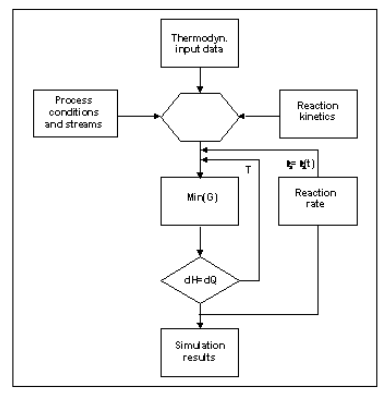Figure 2. The ‘Ratemix’ algorithm used for combination of kinetics with multi-component Gibbs energy calculations2. The algorithm has been used for various metastable and kinetically constrained systems.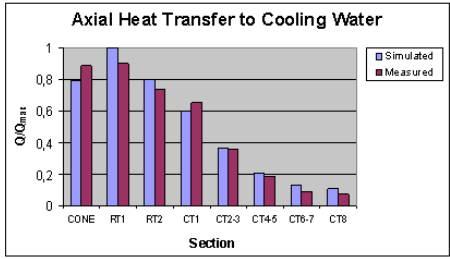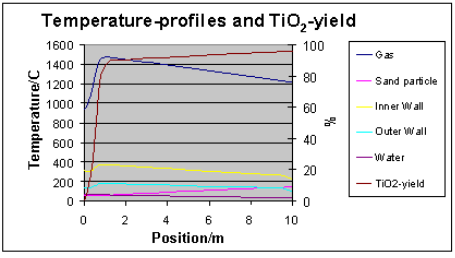Figure 3. The calculated and measured heat transfer of the reactor (upper) and the calculated temperature and conversion profiles for the first 10 m section (lower). The O2:TiCl4-mole ratio was 1.1 and the initial mixing temperature ca. 950 °C. The temperature profile of the scouring sand is included. For measured gas temperatures see ref. 2. The reaction is slightly retarded due to thermodynamic conversion limit at high temperatures. Additional heat transfer completes the reaction. The exotherm can also be utilised for a secondary TiCl4 injection.

Are you interested in more information about how to include kinetic constraints? Have a look at the hydrogen peroxide bleaching process model that includes an aqueous solution based on H2O2-NaOH-O2 where constraints are used to calculate the equilibrium solubility for oxygen, while the hydrogen peroxide is not allowed to decompose to oxygen and water.